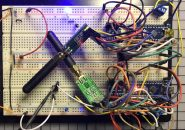## 如何在您的项目中添加LCD显示器

### Arduino扩展板和LCD显示器的工作原理### 所需部件

Arduino UnoLCD显示器扩展板Arduino IDE### 代码

Arduino IDE中自带有一些草图，位于 File > Examples > Liquid Crystal的示例说明中。出于本项目的目的，我们将使用HelloWorld草图。您可以在IDE中加载该草图，以下为该草图的完整代码：

*/

// include the library code:
#include

// initialize the library by associating any needed LCD interface pin
// with the arduino pin number it is connected to
const int rs = 12, en = 11, d4 = 5, d5 = 4, d6 = 3, d7 = 2;
LiquidCrystal lcd(rs, en, d4, d5, d6, d7);

void setup() {
// set up the LCD’s number of columns and rows:
lcd.begin(16, 2);
// Print a message to the LCD.
lcd.print(“hello, world!”);
}

void loop() {
// set the cursor to column 0, line 1
// (note: line 1 is the second row, since counting begins with 0):
lcd.setCursor(0, 1);
// print the number of seconds since reset:
lcd.print(millis() / 1000);
}

#include <LiquidCrystal.h>

const int rs = 12, en = 11, d4 = 5, d5 = 4, d6 = 3, d7 = 2;
LiquidCrystal lcd(rs, en, d4, d5, d6, d7);

void setup() {
// set up the LCD’s number of columns and rows:
lcd.begin(16, 2);
// Print a message to the LCD.
lcd.print(“hello, world!”);
}

void loop() {
// set the cursor to column 0, line 1
// (note: line 1 is the second row, since counting begins with 0):
lcd.setCursor(0, 1);
// print the number of seconds since reset:
lcd.print(millis() / 1000);
}

### 接线### 相关文章

1.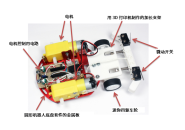#### 在最后一刻停下来！用Arduino和距离传感器制作小鸡赛车！（第3篇•最终篇）

2.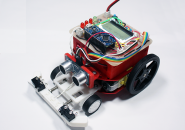#### 在最后一刻停下来！用Arduino和距离传感器制作小鸡赛车！（第2篇）

3.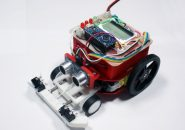#### 在最后一刻停下来！用Arduino和距离传感器制作小鸡赛车！（第1篇）

4.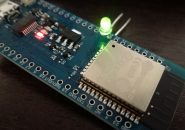#### 通过Arduino使用ESP-WROOM-32（环境搭建和配置-LED闪烁篇）

5.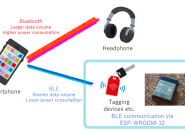#### 通过Arduino使用ESP-WROOM-32（Bluetooth-BLE连接篇）

6.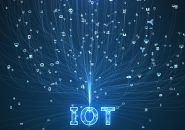#### 六种可以用于扩展物联网应用的DIY项目

7.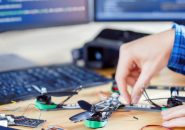#### 使用Arduino的五大DIY无人机项目

8.#### 从电动滑板到无人机：5个DIY项目来提升您的电子技能

9.